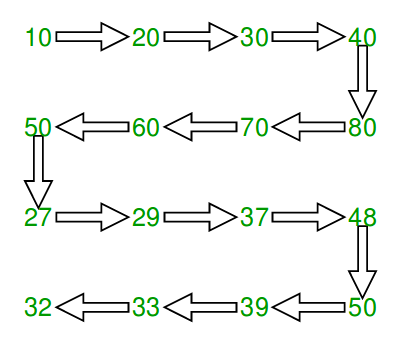GeeksforGeeks App
Open AppBrowser
Continue

# Print matrix in snake pattern

Given an n x n matrix. In the given matrix, you have to print the elements of the matrix in the snake pattern.

Examples :

Input: mat[][] = { {10, 20, 30, 40},

{15, 25, 35, 45},

{27, 29, 37, 48},

{32, 33, 39, 50}};
Output: 10 20 30 40 45 35 25 15 27 29 37 48 50 39 33 32Input: mat[][] = { {1, 2, 3},

{4, 5, 6},

{7, 8, 9}};
Output: 1 2 3 6 5 4 7 8 9

Approach: Follow the steps below to solve the problem:

• Traverse all rows.
• For every row, check if it is even or odd.
• If even, we print from left to right
• else print from right to left.

Below is the implementation of above approach:

## C++

 `// C++ program to print matrix in snake order``#include ``#define M 4``#define N 4``using` `namespace` `std;` `void` `print(``int` `mat[M][N])``{``    ``// Traverse through all rows``    ``for` `(``int` `i = 0; i < M; i++) {` `        ``// If current row is even, print from``        ``// left to right``        ``if` `(i % 2 == 0) {``            ``for` `(``int` `j = 0; j < N; j++)``                ``cout << mat[i][j] << ``" "``;` `            ``// If current row is odd, print from``            ``// right to left``        ``}``        ``else` `{``            ``for` `(``int` `j = N - 1; j >= 0; j--)``                ``cout << mat[i][j] << ``" "``;``        ``}``    ``}``}` `// Driver code``int` `main()``{``    ``int` `mat[M][N] = { { 10, 20, 30, 40 },``                      ``{ 15, 25, 35, 45 },``                      ``{ 27, 29, 37, 48 },``                      ``{ 32, 33, 39, 50 } };` `    ``print(mat);``    ``return` `0;``}`

## Java

 `// Java program to print matrix in snake order``import` `java.util.*;``class` `GFG {``    ``static` `void` `print(``int``[][] mat)``    ``{``        ``// Traverse through all rows``        ``for` `(``int` `i = ``0``; i < mat.length; i++) {` `            ``// If current row is even, print from``            ``// left to right``            ``if` `(i % ``2` `== ``0``) {``                ``for` `(``int` `j = ``0``; j < mat[``0``].length; j++)``                    ``System.out.print(mat[i][j] + ``" "``);` `                ``// If current row is odd, print from``                ``// right to left``            ``}``            ``else` `{``                ``for` `(``int` `j = mat[``0``].length - ``1``; j >= ``0``; j--)``                    ``System.out.print(mat[i][j] + ``" "``);``            ``}``        ``}``    ``}` `    ``// Driver code``    ``public` `static` `void` `main(String[] args)``    ``{``        ``int` `mat[][] = ``new` `int``[][] { { ``10``, ``20``, ``30``, ``40` `},``                                    ``{ ``15``, ``25``, ``35``, ``45` `},``                                    ``{ ``27``, ``29``, ``37``, ``48` `},``                                    ``{ ``32``, ``33``, ``39``, ``50` `} };` `        ``print(mat);``    ``}``}``/* This code is contributed by Mr. Somesh Awasthi */`

## Python3

 `# Python 3 program to print``# matrix in snake order``M ``=` `4``N ``=` `4`  `def` `printf(mat):``    ``global` `M, N` `    ``# Traverse through all rows``    ``for` `i ``in` `range``(M):` `        ``# If current row is``        ``# even, print from``        ``# left to right``        ``if` `i ``%` `2` `=``=` `0``:``            ``for` `j ``in` `range``(N):``                ``print``(``str``(mat[i][j]),``                      ``end``=``" "``)` `        ``# If current row is``        ``# odd, print from``        ``# right to left``        ``else``:``            ``for` `j ``in` `range``(N ``-` `1``, ``-``1``, ``-``1``):``                ``print``(``str``(mat[i][j]),``                      ``end``=``" "``)`  `# Driver code``mat ``=` `[[``10``, ``20``, ``30``, ``40``],``       ``[``15``, ``25``, ``35``, ``45``],``       ``[``27``, ``29``, ``37``, ``48``],``       ``[``32``, ``33``, ``39``, ``50``]]` `printf(mat)` `# This code is contributed``# by ChitraNayal`

## C#

 `// C# program to print``// matrix in snake order``using` `System;``class` `GFG {``    ``static` `void` `print(``int``[, ] mat)``    ``{``        ``// Traverse through all rows``        ``for` `(``int` `i = 0; i < mat.GetLength(0); i++) {` `            ``// If current row is``            ``// even, print from``            ``// left to right``            ``if` `(i % 2 == 0) {``                ``for` `(``int` `j = 0; j < mat.GetLength(1); j++)``                    ``Console.Write(mat[i, j] + ``" "``);` `                ``// If current row is``                ``// odd, print from``                ``// right to left``            ``}``            ``else` `{``                ``for` `(``int` `j = mat.GetLength(1) - 1; j >= 0;``                     ``j--)``                    ``Console.Write(mat[i, j] + ``" "``);``            ``}``        ``}``    ``}` `    ``// Driver code``    ``public` `static` `void` `Main()``    ``{``        ``int``[, ] mat = { { 10, 20, 30, 40 },``                        ``{ 15, 25, 35, 45 },``                        ``{ 27, 29, 37, 48 },``                        ``{ 32, 33, 39, 50 } };` `        ``print(mat);``    ``}``}` `// This code is contributed``// by ChitraNayal`

## PHP

 `= 0; ``\$j``--)``                ``echo` `\$mat``[``\$i``][``\$j``] , ``" "``;``        ``}``    ``}``}` `// Driver code``\$mat` `= ``array``(``array``(10, 20, 30, 40),``             ``array``(15, 25, 35, 45),``             ``array``(27, 29, 37, 48),``             ``array``(32, 33, 39, 50));` `printLN(``\$mat``);` `// This code is contributed by ajit``?>`

## Javascript

 ``

Output

`10 20 30 40 45 35 25 15 27 29 37 48 50 39 33 32 `

Time Complexity: O(N x M), Traversing over all the elements of the matrix, therefore N X M elements are there.
Auxiliary Space: O(1)

This article is contributed by Rakesh Kumar. If you like GeeksforGeeks and would like to contribute, you can also write an article using write.geeksforgeeks.org or mail your article to review-team@geeksforgeeks.org. See your article appearing on the GeeksforGeeks main page and help other Geeks.

My Personal Notes arrow_drop_up